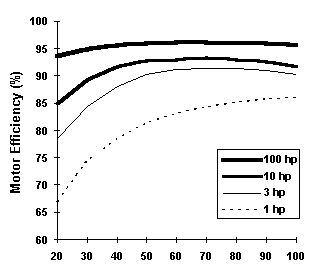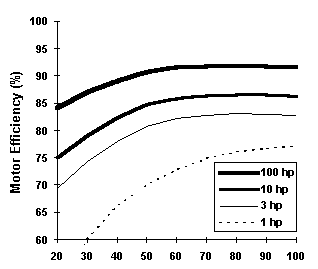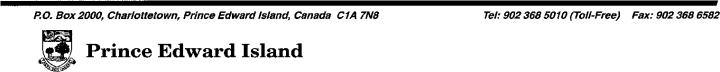February  1996                                                                                                                                   Volume 3   Issue 4 Measuring Motor Performance     Those familiar with motors know that just because a motor has a nameplate rating of 50 horsepower doesn’t mean that it actually delivers 50 horsepower to its load.  The load itself determines the horsepower needed to drive it, and whatever motor is connected to that load will try to deliver the power that the load demands.  If the motor is called upon to deliver more power than it is designed to deliver, it will surely overheat, possibly stall, and eventually burn out.  If it is called upon to deliver much less than rated power, it will operate inefficiently, wasting energy and energy dollars.      As pointed out in earlier articles in this series, knowing how much power each of your plant’s large motors is being called upon to deliver is an important part of an effective energy-reduction strategy.  Spotting overloaded motors can help you avoid motor burnouts.  Spotting underloaded motors can lead to downsizing and reduced operating costs.  And as pointed out two issues ago (Vol. 3 No. 2), load factor profiles can help you determine if (and where) adding adjustable speed drives (ASDs) would be cost effective.  The present article tells how to acquire these important load factor and output power numbers. LOAD FACTOR (LF)     The ratio of the actual power coming out of a motor to its rated power is called the motor’s load factor — LF.  It is usually expressed in per cent:         100 × Actual Power Out LF (%) = ————————————           Rated Power Out Of course, if you happen to know the load factor, you can calculate the Actual Power Out:                LF (%) x Rated Power Out Actual Power Out = ————————————–                        100 DETERMINING MOTOR LOAD FACTOR     There is no way of knowing, just by glancing at an operating motor, how much power it is currently delivering to its load.  But there are two ways of getting this information.  The first (preferred) way calls for measuring the electrical input power to the motor.  The second calls for measuring the motor’s exact shaft speed under load.  Power Input Method     The relationship between load factor and motor power input in kilowatts (kW) is:      Input Power (kW) x Efficiency (%) LF (%) = —————————————————           Rated Horsepower x 0.746 where Efficiency is the per cent efficiency of the motor at that load factor.  The input power should be measured using a wattmeter of the “true RMS” type.  In situations where the motor load varies, it is helpful to measure input power for a day or more using a recording wattmeter.  (The Energy and Minerals Section has one of these instruments.  For further information, call Ron Estabrooks or Mike Proud at 368-5010, toll free.)    The second part of the puzzle involves coming up with an estimate of motor efficiency.  The exact efficiency is almost never known, but there are several ways to arrive at a reasonable approximation.    1.      Refer to the motor manufacturer’s literature or motor nameplate data. 2.      Refer to a motor-specification database such as the one included in the MotorMaster software.  Some electric utilities make such data available to customers. 3.      Use typical efficiency information such as that presented in the two graphs, or a combination of typical curves and single-point actual data.     Note that to select an efficiency figure for the load factor (LF) equation you need to make an assumption beforehand about what the load factor is likely to be.  Start with your best guess, run the calculation, then use the resulting LF to arrive at a better efficiency estimate.  Finally, plug that new Efficiency number into the equation, and perform the calculation again. Shaft Speed Method     The other way to determine motor load factor is to measure motor shaft speed under load using an accurate strobe tachometer.        Synchronous Speed - Actual Speed LF (%) = —————————————————— x 100        Synchronous Speed - Rated Speed For example, if the motor has a synchronous speed* of 1800 rpm, a rated “nameplate” speed of 1740 rpm, and an actual speed of 1770 rpm, the load factor will be:         1800 - 1770 LF (%) = ———————— x 100 = 50%         1800 - 1740 The term slip refers to the difference between synchronous speed and actual speed.  In the equation above, the numerator is operating slip and the denominator is full-load slip.     The tachometer method is usually faster and easier than the wattmeter method.   Assuming an accurate tachometer, the method would be accurate if the Rated Speed on the motor nameplate represented the exact speed at which full load power is delivered.  Unfortunately, the number on the nameplate is just an approximation, usually rounded off to the nearest 5 rpm and subject to further error due to testing tolerances and differences in motor voltage.  Naturally, if you take measurements using both techniques and they agree, the tachometer can be used from then on to check the performance of that particular motor.   * The synchronous speed of an induction motor equals 7200 divided by the number of poles the motor has.  Synchronous speed will always be a few percentage points faster than rated speed, with the most common values being 3600, 1800, 1200, 900, and 600 rpm.   TYPICAL EFFICIENCY PROFILES — SEVERAL 1800 RPM 3-PHASE MOTORS                Energy-Efficient Models                           Standard-Efficiency Models